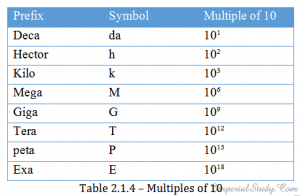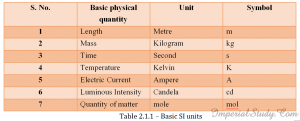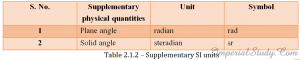# Science | Class 11th Notes | Physics | Unit – 1 Physical World and Measurements Chapter – 2 Systems of Units (Part – I)

0
2015We provide students with the best science notes for class 11th that helps to repack the long and lengthy course into a short series of easy notes that reduces the burden from the shoulders of students regarding heavy course and hard study and shows them a new and easy way to study. Until class 10th, students were taught in a lengthy, easy and explained method so that their basic knowledge about science gets strong. Class 11th is about expanding that basic knowledge and have got a very lengthy course which is many times more than that of 9th and 10th class. Also, if a student seeks to go into competitions like I.I.T., A.I.E.E.E., A.I.M.M.S. than the load of study he needs to do is much more increased. This load can be decreased if he is presented his vast course in form of short and easy notes which the students can easily understand and learn. That is why we have prepared the whole large and vast course for Class 11th in form of short and easy notes for our students. You can read them and find it out for yourself how easy is to read and learn them. We would appreciate your feedbacks or suggestions in form of comments and if there is something still missing, we will be keeping updating the post for you. You must keep visiting the website to get those updates.

In the second chapter of first unit, we come across a problem. A large number of topics is to be covered in the second chapter, each topic having specific type of questions, problems and numericals associated with them. Fitting all those topics in one chapter would make it difficult to understand and the chapter will become really bulky. So, we have divided the second chapter into four parts, ‘Units and quantities’, ‘Length, mass and time measurement’, ‘Dimensional Analysis’ and ‘Error Analysis’. This would help the students to understand all the topics in four different parts and making them easier to understand and learn.

Science for class – XI

Unit -1: Physical world and measurements

Although we have been studying the physics from a long time, still there is not everything we have been able to clear out in our previous classes. We need to revisit the world of physics with a new dimension and explore the basics of physics and physical study again so that nothing remains uncovered and no single basic concept of physics is left. In unit Ist i.e. Physical world and measurements, we look at all the basic knowledge of physics so that armed with this knowledge we can move onto further deep study of physics in further units.

Chapter – 2: Systems of Units

Part-1: Units and Quantities

2.1.1 – Introduction

In this first part of chapter two, we will discuss the topics surrounding units and quantities. We would discuss about some earlier definitions of some common units and then introduce the SI system of units and how it defines the basic and supplementary units.

2.1.2 – What are units?

Before we move on to units, let us talk about physical quantities.

Physical quantity: All quantities in terms of which laws of physics can be explained and which can be measured directly or indirectly are physical quantities. For example length, mass, time etc.

Now let us move to units.

Units: The standard that is chosen as reference in order to measure a physical quantity is called the unit of that quantity. For example, the unit of length which is metre, or the unit of mass which is kilogram.

Process of measurement of physical quantities:

1. The selection of the unit.
2. Determining the number of times the unit is contained in that physical quantity.

For example, in the first step, we select metre as the unit of length. Next, in the second step, we measure the length of a room. To do so, we determine how many metres are contained in the room we are measuring. We know that the room’s length can be measured using various methods and we usually say that the room is 7 metres or 8 metres.

Measure of a physical quantity = Numerical value of the physical quantity × Size of its unit

X = n u

It follows that if the size of the unit is small, numerical value of the quantity will be large and vice-versa.

n u = constant

If n1 = numerical value of physical quantity of unit u1.

n2 = numerical value of physical quantity of unit u2.

n1 u1 = n2 u2

2.1.3 – Fundamental and Derived Units.

As the title says, in this topic we would discuss about two different categories of units.

Fundamental units: Those units which are neither derived from other units, nor can be further resolved into other units are known as fundamental units.

Mass, length and time are fundamental quantities and their units are called fundamental units.

Derived units: Those units which are derived from other fundamental units are known as derived units.

Area is a derived unit. If the side of a square is ‘x’ metres, then its area is x × x = x2 metres.

The unit of any physical quantity can be derived from its defining equation. e.g.:

Speed = Distance/Time

Unit of speed = Unit of length/Unit of time

= metre/second

=m s-1

2.1.4 – Standard Units.

A unit that is most appropriate or most suitable for a quantity/physical quantity is called a standard unit.

Characteristics of a standard unit:

1. It should be well-defined.
2. It should be of suitable size i.e. neither too large nor too small.
3. It should be easily reproducible at all places.
4. It should not change with time and from place to place.
5. It should not change with change in physical properties.
6. It should be easily accessible.

2.1.5 – Some physical units and their earlier definitions

Unit of mass:

Mass: Mass of a body is the quantity of matter it contains.

Mass of a material body can never be zero. The internationally accepted unit of mass is kilogram.

Unit of length:

Length: Length of an object is the distance of separation between its two ends.

Internationally accepted unit of length is metre.

Unit of time:

Time: It is not possible to define time in absolute terms. However, according to Einstein, time is simply what a clock reads.

Internationally accepted unit of time is second.

Earlier definitions of these units:

Mass:

1. Originally, one kilogram was defined as the mass of one cubic decimetre of water at 4° (Temperature of water at maximum density)
2. The General Conference of Weights and Measures defined one kilogram as the mass of a platinum-iridium cylinder kept at the International Bureau of Weights and Measures at Sevres, near Paris, France.

Length:

1. In 1971, the Paris Academy of Sciences defined metre as one-tenth millionth of the distance from north pole to equator.
2. In 1899, the General Conference of Weights and Measures defined metre as the distance between two lines marked on a platinum-iridium rod kept at a constant temperature of 273.16 K preserved at International Bureau Of Weights And Measures.

There are two reasons:

1. If the temperature of the rod changes, its length will change too.
2. It will be difficult to compare any metre rod or a newly produced rod with the preserved rod.

Time:

1. Paris Academy of Sciences defined one second as the time taken by a simple pendulum of one metre to swing between two extreme points.
2. The time when sun is at the highest point is called noon. Solar day is the time that elapses between noons of two consecutive days.

Mean solar day is the average of all solar days in one year. And a solar second is the (1/24 × 60 × 60)th of a mean solar day.

Duration or length of a mean solar day is different for different years. So, this definition was not appropriate.

Q – What difficulties did earlier units of mass and length did present?

Some difficulties were:

1. It was difficult to preserve kilogram and a metre bar.
2. It was difficult to replicate them for their use in different countries.
3. It was difficult to compare the replicas with the preserved kilogram and metre bar.

2.1.6 – International systems of units.

1. cgs system :
• French system of units.
• Uses centimetre, gram and second as basic units.
• It is a metric system of units.

2.fps system :

• British/English system of units.
• Uses foot, pound and second as basic units.
• It is not a metric system of units.

3.mks system :

• Also a French system of units.
• Uses metre, kilogram and second as basic units.
• A metric system of units. Closely related to cgs system.
• A coherent system of units.

Coherent system of units: If all derived units can be obtained by either multiplying or dividing its fundamental units, such that no numerical factors are introduced.

4.SI : The General Conference of Weights and Measures held in 1960 introduced a new logical system of units known as Systeme Internationale d’ Unites or SI in short.

• Redefines units on the basis of atomic standards.
• Covers all the branches of physics.
• Based on seven basic and two supplementary units.

Following is the table of the seven basic units defined by SI:Following is the list of supplementary SI units:2.1.7 – Basic and supplementary SI units.

Basic units:

Metre: According to General Conference of Weights and Measures, one metre equals to 1,650,763.3 wavelengths in the vacuum of orange-red radiation emitted by Krypton with atomic mass 86.

In 1983, metre was redefined as length of path travelled by light in vacuum during a time interval of 1/299,792,458 of a second.

Kilogram : It was not redefined on atomic standards. So, one kilogram is the mass of the platinum-iridium cylinder kept at the International Bureau of Weights and Measures in Sevres, near Paris, France.

Second : In 1964, the twelfth General Conference of Weights and Measures defined second as equal to the duration of 9,192,631,770 vibrations corresponding to the transition between two hyperfine levels of cesium-133 atom in the ground state.

Kelvin : It is defined as 1/273.16th fraction of the thermodynamic temperature at the triple point of water.

Ampere : One ampere is defined as the current generating a force of 2 ×10-7 newton per metre square between two parallel straight conductors of infinite length and negligible circular cross-section, when placed one metre apart in vacuum.

Candela : One candela is the luminous intensity in the perpendicular direction of a surface of 1/6,00,000 square metre of a black body at a temperature of freezing platinum (2046.65 K) and under a pressure of 1,01,325 newton per metre square.

In 1979, candela was redefined as the luminous intensity in a given direction due to a source which emits monochromatic radiation of frequency of 540 × 1012 Hz and of which the radiant intensity in that direction is 1/683 watt per steradian.

Mol : One mol was defined as the amount of substance having the same number of elementary particles as there are atoms in 0.012 kg of carbon-12.

Supplementary SI units:

Radian : It is the plane angle between two radii of a circle, which cut off from the circumference, an arc equal to the length of the radius of the circle.

Steradian : It is the solid angle with its apex at the centre of a sphere, which cuts out an area on the surface of the sphere equal to the area of an square whose sides are equal to the radius of the circle.

solid angle (in steradian) = area cut out from the surface of the sphere/radius2

1. It is a rational system of units : It makes use of only one unit for one physical quantity while other systems may use different units for a single quantity.
2. SI is a coherent system of unites : All derived units can be obtained by dividing and multiplying basic and supplementary units and no numerical factors are introduced, while in other systems, numerical factors may be introduced.
3. Closely related to cgs system : It is very easy to convert cgs units into SI units or vice-versa.
4. SI is a metric system : Like cgs and mks, SI is also a metric system of units. All multiples and submultiples can be expressed as the powers of 10.

2.1.8 – Some prefixes in power of 10.

Submultiples:

Multiples :

Some side-notes :

The following units of lengths are used for measuring very small units:

1. 1 fermi/femtometre (fm) = 10-15 m
2. 1 angstorm = 10-10 m
3. 1 micron/micrometre = 10-6 m

The following units are used for measuring very large distances:

Light year : Distance travelled by light in vacuum in one year.

1 light year = 9.46 × 1015 m

Astronomical unit (AU) : Mean distance of the sun from the earth.

1 AU = 1.496 × 1011 m

Parallactic second (parsec) : Distance at which an arc of length one AU subtends an angle of one second of an arc.

1 parsec = 3.08 × 1016 m

Atomic mass unit:

In atomic and nuclear physics,, mass is measured in terms of atomic mass unit (a.m.u.).

One a.m.u. is defined as 1/12th of the mass of one Carbon-12 atom.

1 a.m.u. = 1.66 × 10-27 kg.